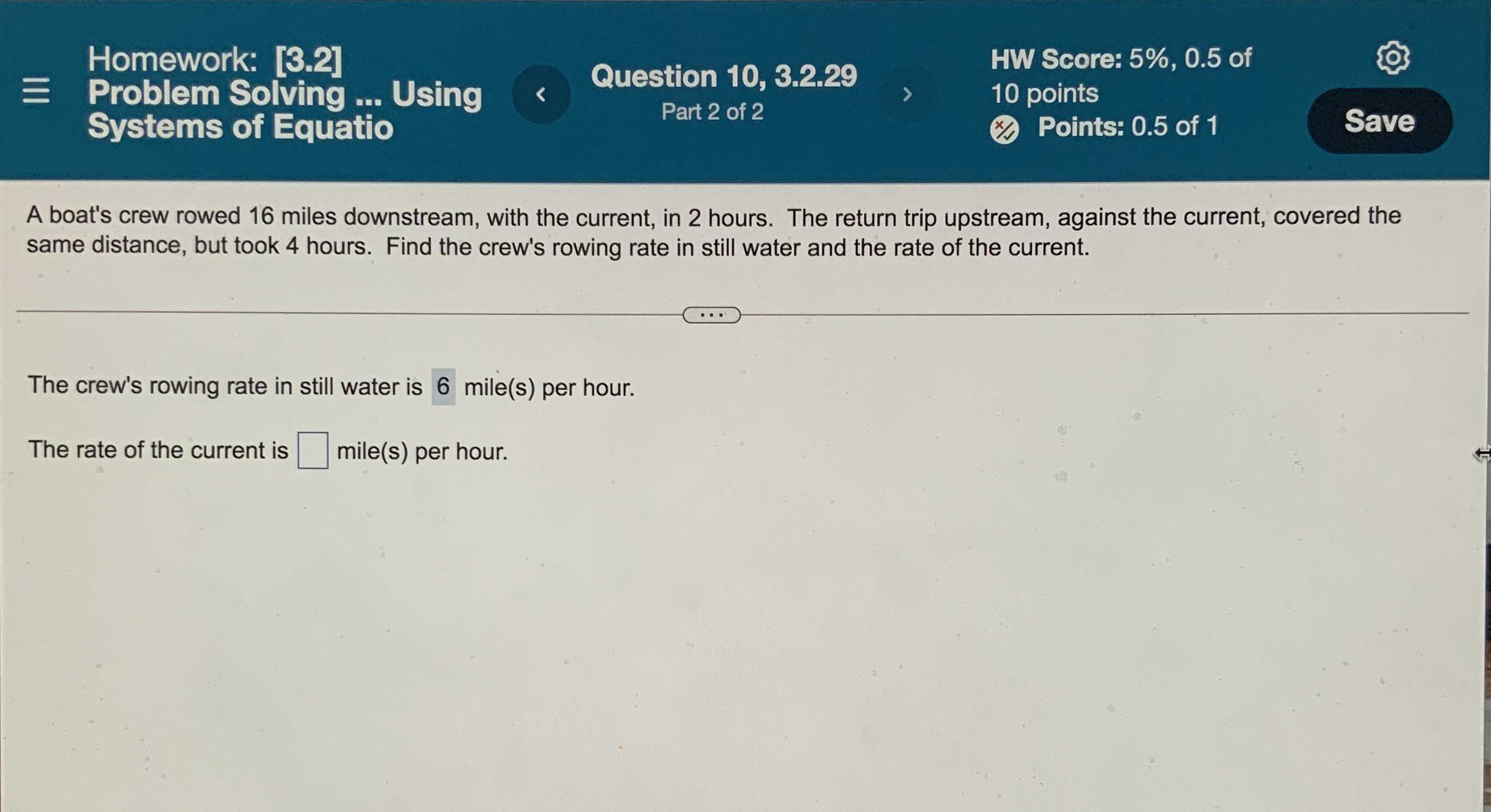### ¿Todavía tienes preguntas de matemáticas?

Pregunte a nuestros tutores expertos
Algebra
PreguntaA boat's crew rowed $$16$$ miles downstream, with the current, in $$2$$ hours. The return trip upstream, against the current, covered the same distance, but took $$4$$ hours. Find the crew's rowing rate in still water and the rate of the current.

The crew's rowing rate in still water is $$\square$$ mile(s) per hour.

The rate of the current is $$\square$$ mile(s) per hour.